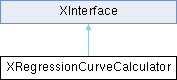LibreOffice LibreOffice 7.4 SDK API Reference
XRegressionCurveCalculator Interface Reference

`import"XRegressionCurveCalculator.idl";`

Inheritance diagram for XRegressionCurveCalculator:## Public Member Functions

void setRegressionProperties ([in] long degree, [in] boolean forceIntercept, [in] double interceptValue, [in] long period, [in] long movingType)
set calculation properties for curve calculation. More...

void recalculateRegression ([in] sequence< double > aXValues, [in] sequence< double > aYValues)
recalculates the parameters of the internal regression curve according to the x- and y-values given. More...

double getCurveValue ([in] double x) raises ( com::sun::star::lang::IllegalArgumentException )
calculates the value of the regression curve for x. More...

sequence< com::sun::star::geometry::RealPoint2DgetCurveValues ([in] double min, [in] double max, [in] long nPointCount, [in] XScaling xScalingX, [in] XScaling xScalingY, [in] boolean bMaySkipPointsInCalculation) raises ( com::sun::star::lang::IllegalArgumentException )
calculate multiple points of a regression curve at once. More...

double getCorrelationCoefficient ()
Returns the value of the correlation coefficient for the given regression. More...

string getRepresentation ()
Retrieve a string showing the regression curve's function with calculated parameters. More...

string getFormattedRepresentation ([in] com::sun::star::util::XNumberFormatsSupplier xNumFmtSupplier, [in] long nNumberFormatKey, [in] long nFormulaLength)
Returns a representation using the given number format for formatting all numbers contained in the formula. More...

void setXYNames ([in] string aXName, [in] string aYName)
Set the names of X and Y variables of the equation to replace "x" and "f(x)" in representation. More...Public Member Functions inherited from XInterface
any queryInterface ([in] type aType)
queries for a new interface to an existing UNO object. More...

void acquire ()
increases the reference counter by one. More...

void release ()
decreases the reference counter by one. More...

## ◆ getCorrelationCoefficient()

 double getCorrelationCoefficient ( )

Returns the value of the correlation coefficient for the given regression.

This value is often denoted as r or R.

The value of r is signed. Often r2 is used instead of r to denote a regression curve's accuracy.

Returns
The return value is the fraction of the variance in the data that is explained by the regression.

## ◆ getCurveValue()

 double getCurveValue ( [in] double x ) raises ( com::sun::star::lang::IllegalArgumentException )

calculates the value of the regression curve for x.

Parameters
 x The abscissa value for which the value of the regression curve should be calculated. All numbers that are part of the domain of the regression function are valid.
Returns
If x is element of the domain of the regression curve function, the result is its value.
Exceptions
 com::sun::star::lang::IllegalArgumentException If x is not part of the domain of the regression function.

## ◆ getCurveValues()

 sequence< com::sun::star::geometry::RealPoint2D > getCurveValues ( [in] double min, [in] double max, [in] long nPointCount, [in] XScaling xScalingX, [in] XScaling xScalingY, [in] boolean bMaySkipPointsInCalculation ) raises ( com::sun::star::lang::IllegalArgumentException )

calculate multiple points of a regression curve at once.

Note that this method may optimize the output by returning less points, e.g. for a line you may get only two resulting points instead of nPointCount() points. This is only allowed if the parameter bMaySkipPointsInCalculation() is set to `TRUE`.

It is important that a renderer takes the scalings into account. When one of these parameters is unknown, no optimization must be done.

Parameters
 min the abscissa value for the starting point. max the abscissa value for the ending point. nPointCount the number of points to calculate. bMaySkipPointsInCalculation determines whether it is allowed to skip points in the calculation. When this parameter is `TRUE` it is assumed that the underlying coordinate system is Cartesian. xScalingX a scaling that is used for the values in x-direction xScalingY a scaling that is used for the values in y-direction

## ◆ getFormattedRepresentation()

 string getFormattedRepresentation ( [in] com::sun::star::util::XNumberFormatsSupplier xNumFmtSupplier, [in] long nNumberFormatKey, [in] long nFormulaLength )

Returns a representation using the given number format for formatting all numbers contained in the formula.

Wrap equation to fit in nFormulaLength characters

getRepresentation

## ◆ getRepresentation()

 string getRepresentation ( )

Retrieve a string showing the regression curve's function with calculated parameters.

Returns
The string returned contains the regression curve's formula in a form
`"f(x) = ..."`
, where the calculated parts are filled out. For a linear regression you might get
`"f(x) = 0.341 x + 1.45"`
.

## ◆ recalculateRegression()

 void recalculateRegression ( [in] sequence< double > aXValues, [in] sequence< double > aYValues )

recalculates the parameters of the internal regression curve according to the x- and y-values given.

Parameters
 aXValues All x-values that represent the measurement points on which the regression is based aYValues All y-values that represent the measurement points on which the regression is based

## ◆ setRegressionProperties()

 void setRegressionProperties ( [in] long degree, [in] boolean forceIntercept, [in] double interceptValue, [in] long period, [in] long movingType )

set calculation properties for curve calculation.

Parameters
 degree Degree of polynomial regression curve, value should be greater than zero If the curve is not polynomial, this property has no effect. period Period of a moving average regression curve, value should be greater or equal to 2 If the curve is not moving average regression curve, this property has no effect. forceIntercept Should force the intercept value. interceptValue Intercept value. movingType Only if regression type is "Moving Average"